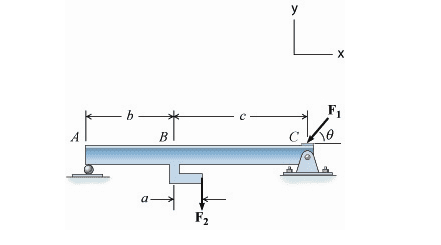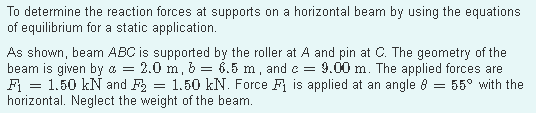# Help finding the vertical component of the reaction at C

Homework Statement:
Finding the vertical component of the reaction at C
Relevant Equations:
E of EHello everyone,

For this equation, I have found Ay as 0.677kN by using Ma = 1.5kN(7m)-Ay(15.5m).

To find Cy, would I be using the sum of forces to find the answer?

For example Fy = Ay - F2 - F1sin55 + Cy = 0
This would then get Cy answer as 2.05kN

## Answers and Replies

haruspex
Science Advisor
Homework Helper
Gold Member
2020 Award
This would then get Cy answer as 2.05kN
Looks right.

Steve4Physics
Homework Helper
Gold Member
Hi. Method is fine but pay attention to detail.
E.g. Don't use 'a' for 'A' (as in 'Ma'). Don't forget to say MA equals 0. Personally I would just say 'moments about A' rather than 'MA'.
For this equation, I have found Ay as 0.677kN by using Ma = 1.5kN(7m)-Ay(15.5m).
So it would be clearer to write the equation as (for example):
1.5kN(7m) - Ay(15.5m) = 0 (moments about A)

I would use one or two additional significant figures for intermediate-step answers (e.g. Ay = 0.6774kN). This reduces accumulated rounding errors in later steps. Round final answers as appropriate at the end.